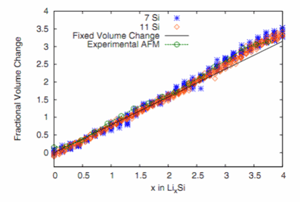# First‑principles studies of the reaction of Li with amorphous Si

When lithium reacts with amorphous silicon, amorphous LixSi is formed. The voltage composition curve of Li/a-LixSi cells has been calculated using density functional theory (DFT) as implemented in ABINIT1. This represents the first such calculation for an amorphous system. Reference 1 has 31 citations as of Nov. 22, 2012.Figure 1

The calculation is centered between the experimental charge and discharge curves.Figure 2 The calculated volume changes match the experimental volume changes.

#### References

1. V.L. Chevrier and J.R. Dahn, First Principles Model of Amorphous Silicon Lithiation, J.Electrochem. Soc. 156, A454-A458 (2009).